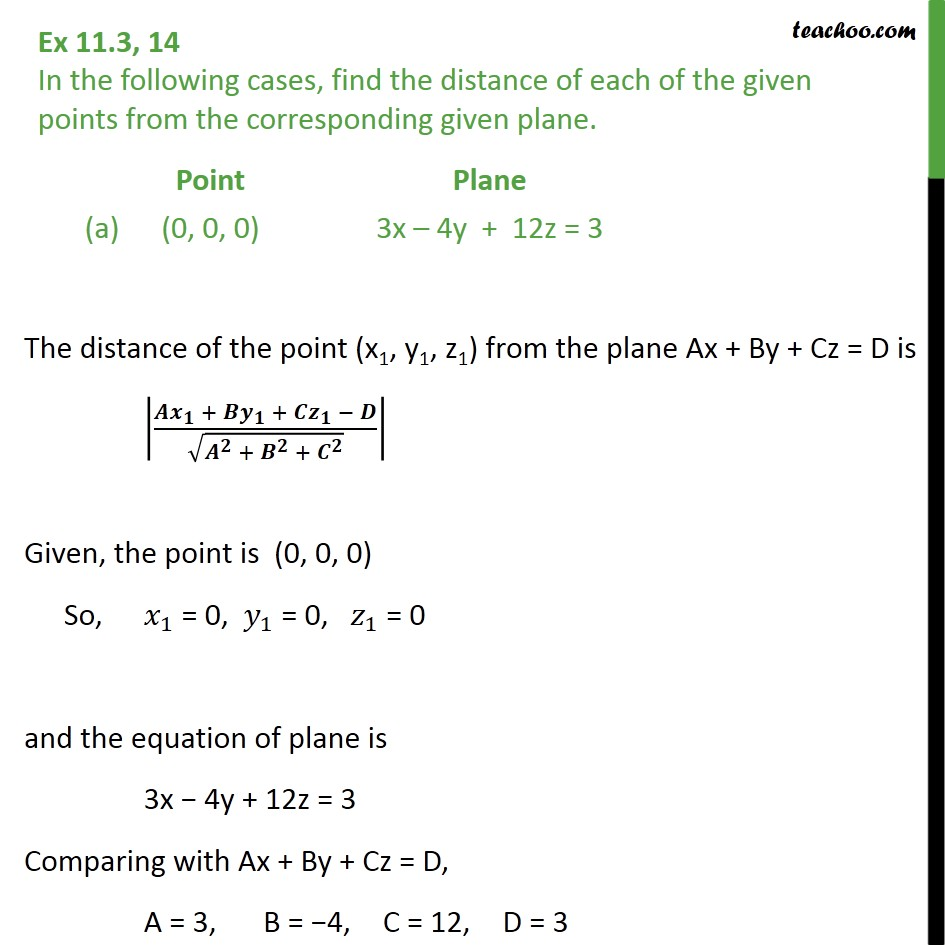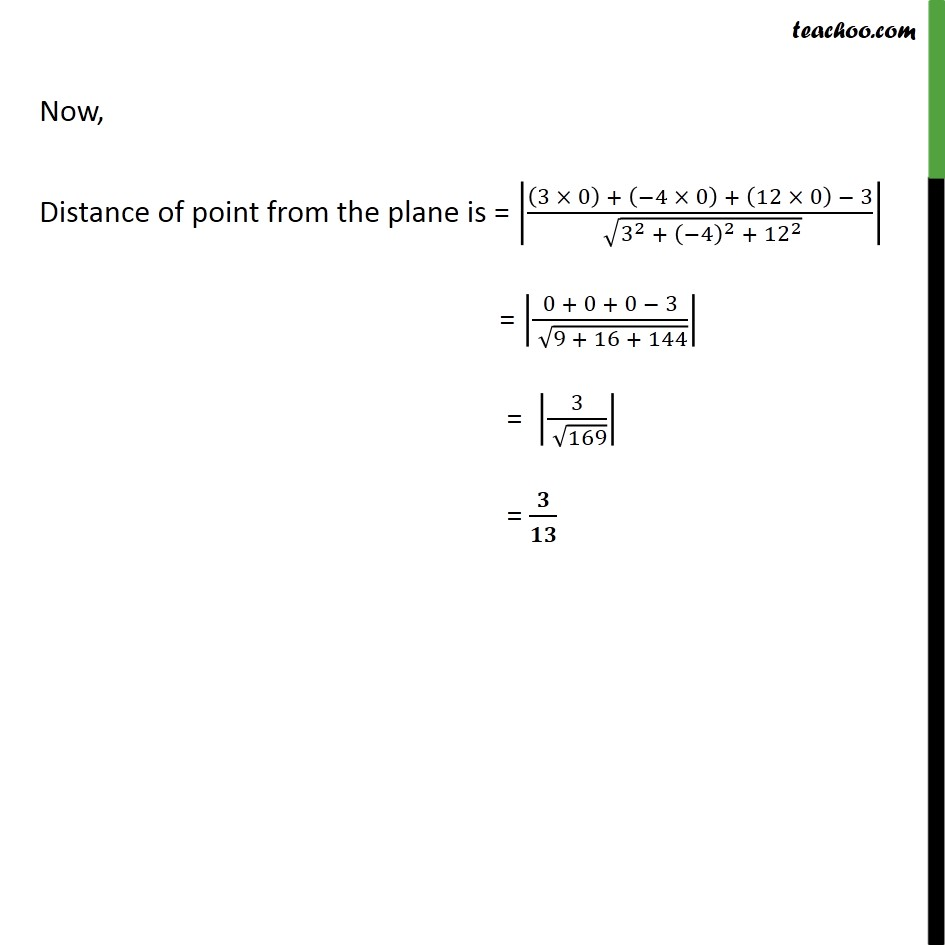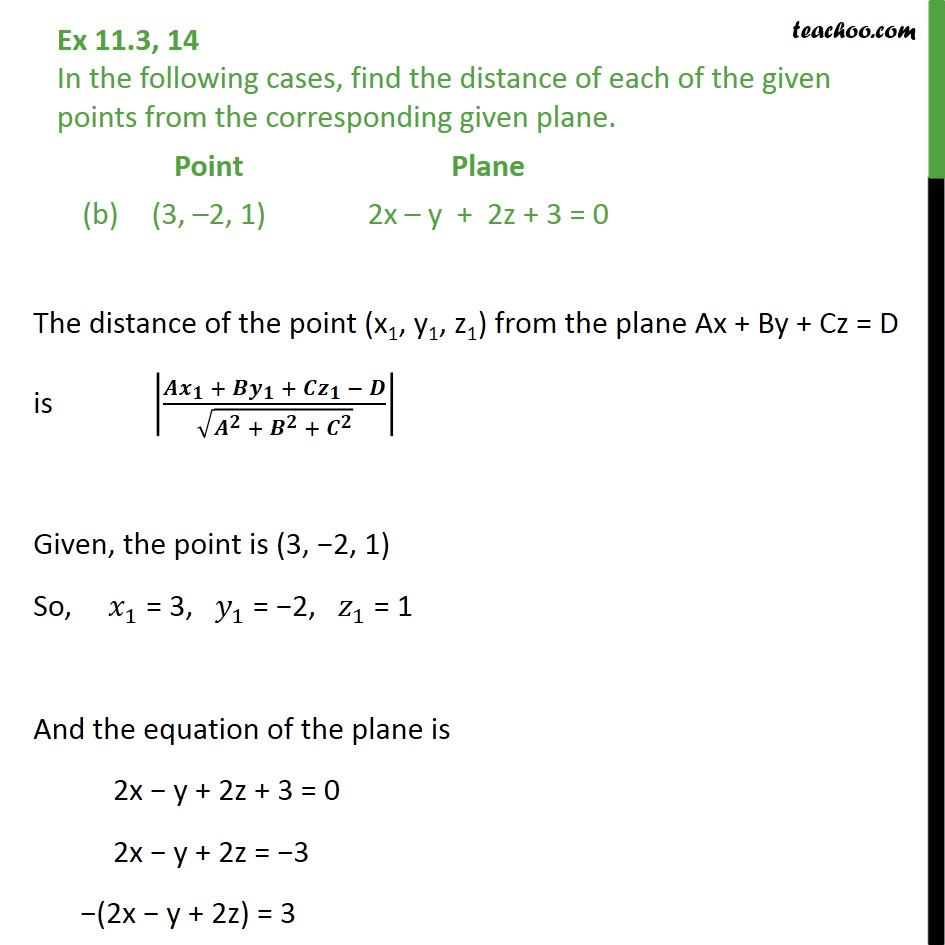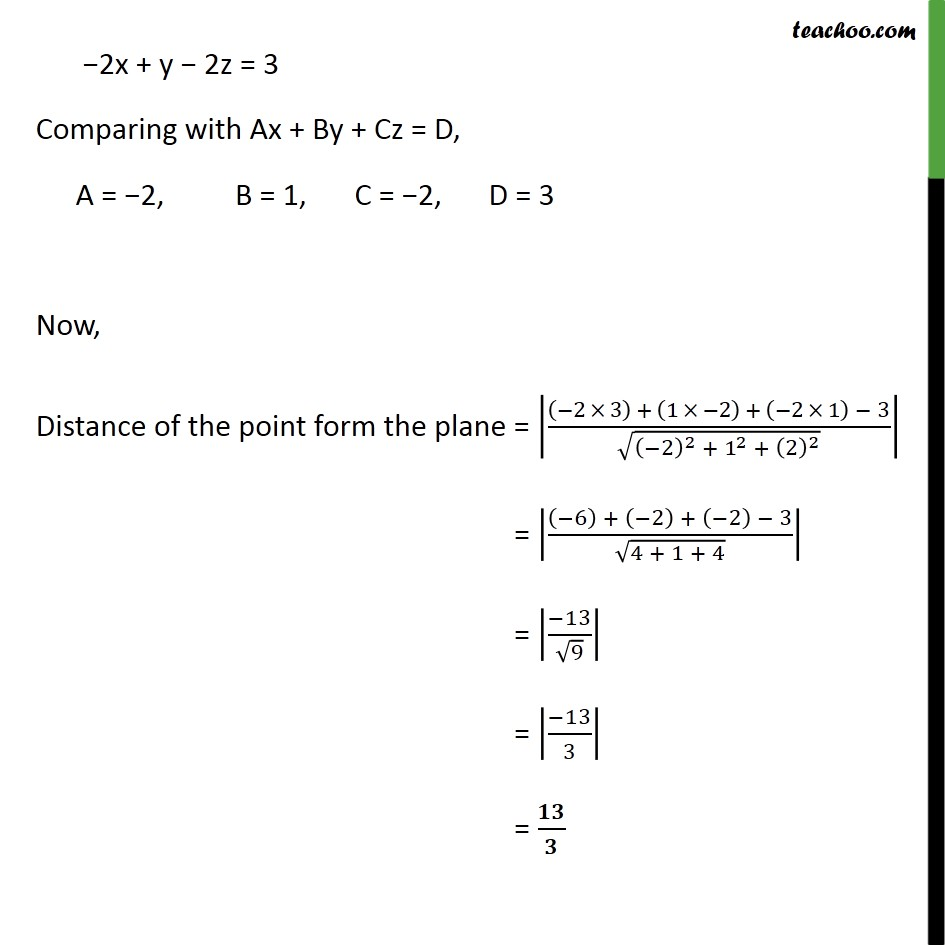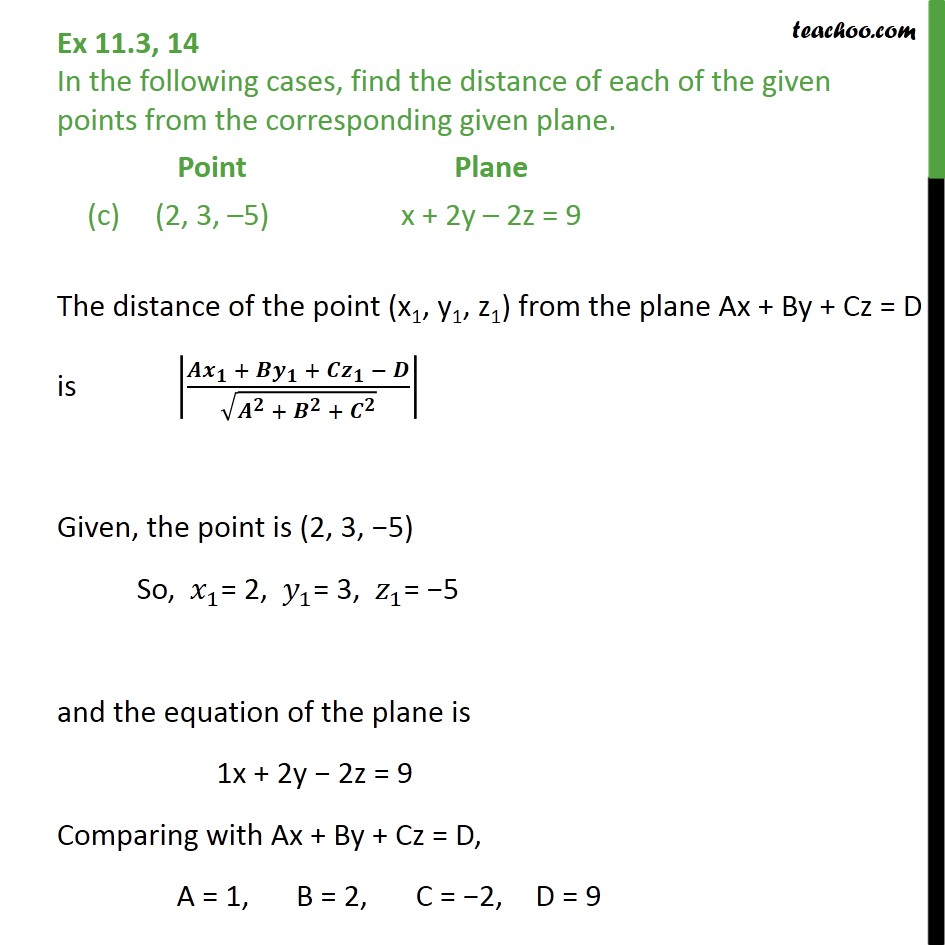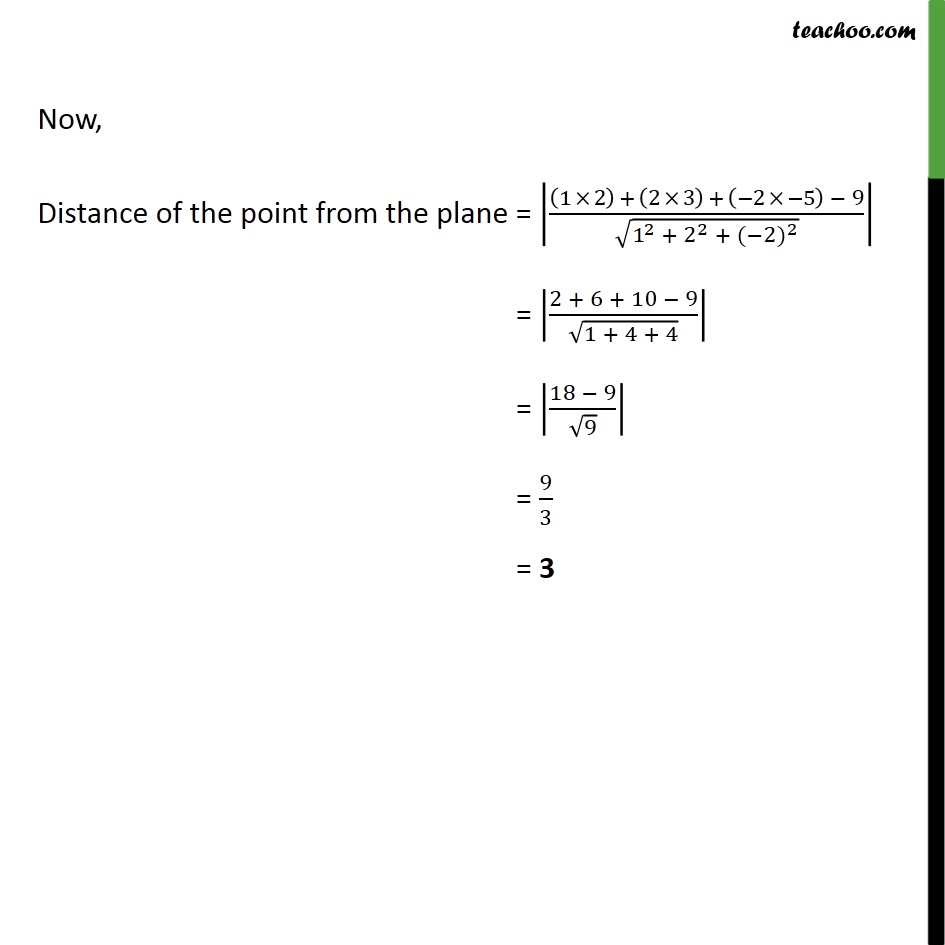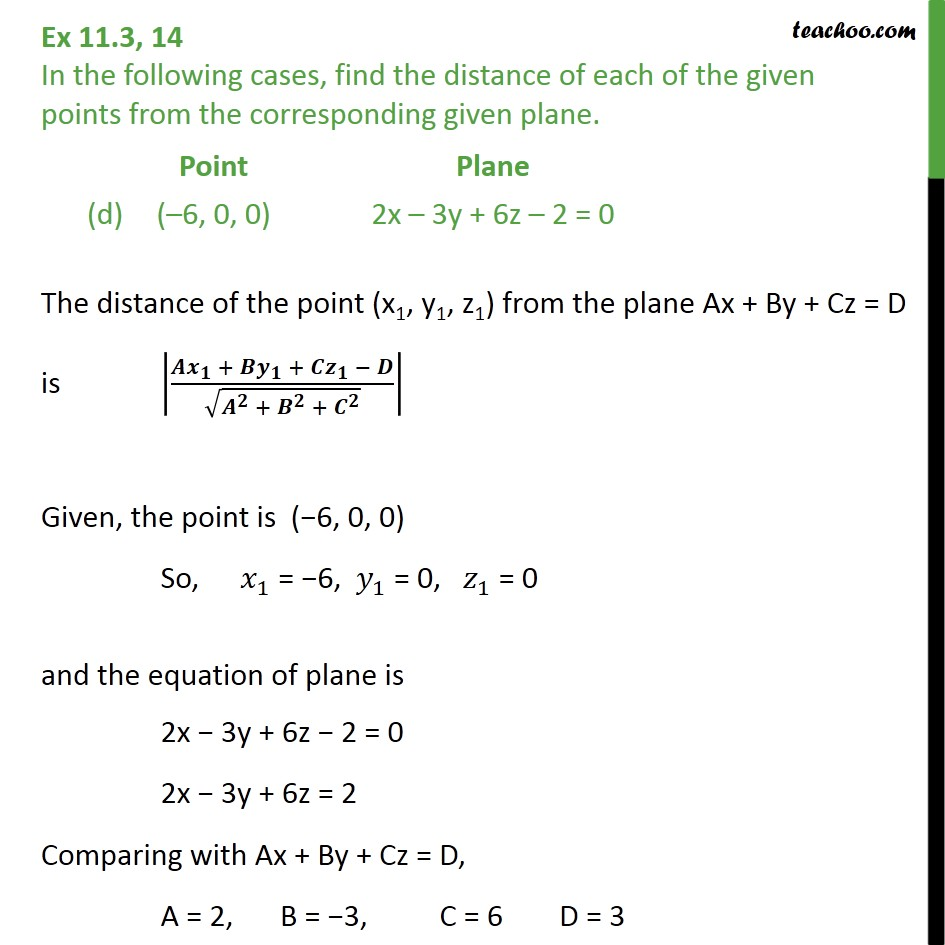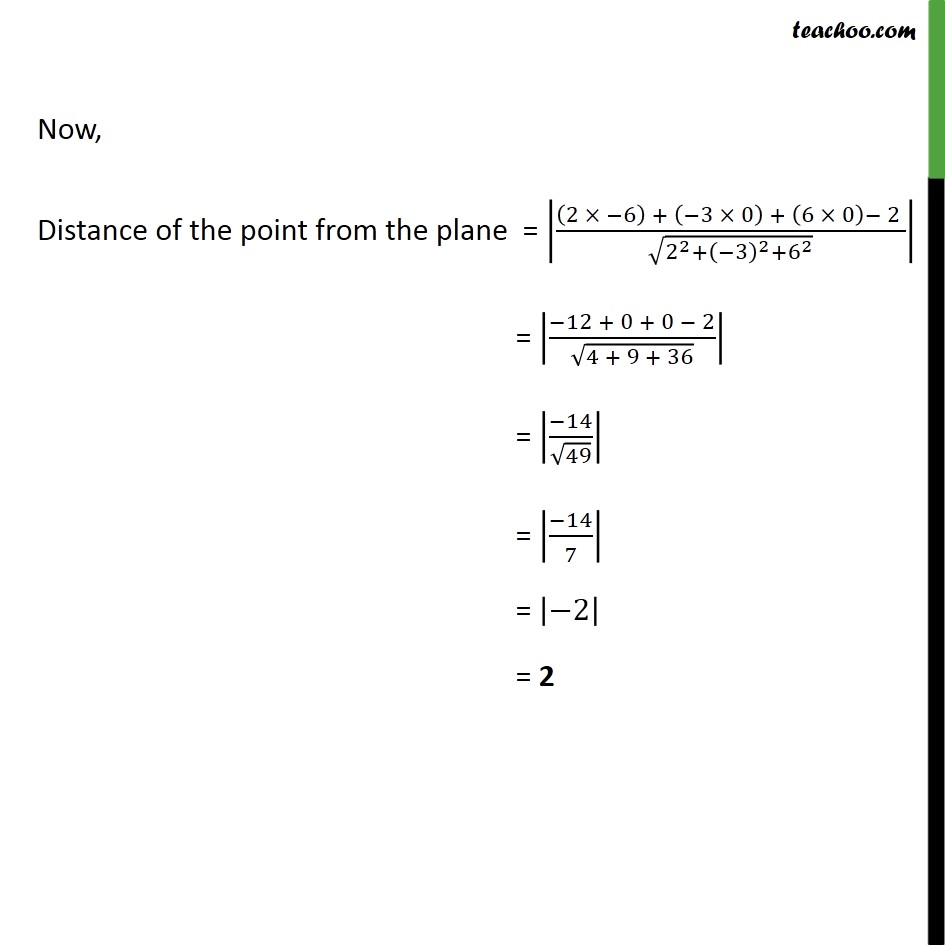1. Chapter 11 Class 12 Three Dimensional Geometry
2. Serial order wise
3. Ex 11.3

Transcript

Ex 11.3, 14 In the following cases, find the distance of each of the given points from the corresponding given plane. The distance of the point (x1, y1, z1) from the plane Ax + By + Cz = D is 𝑨 𝒙﷮𝟏﷯ + 𝑩𝒚﷮𝟏﷯ + 𝑪𝒛﷮𝟏﷯ − 𝑫﷮ ﷮ 𝑨﷮𝟐﷯ + 𝑩﷮𝟐﷯ + 𝑪﷮𝟐﷯﷯﷯﷯ Given, the point is (0, 0, 0) So, 𝑥﷮1﷯ = 0, 𝑦﷮1﷯ = 0, 𝑧﷮1﷯ = 0 and the equation of plane is 3x − 4y + 12z = 3 Comparing with Ax + By + Cz = D, A = 3, B = −4, C = 12, D = 3 Now, Distance of point from the plane is = 3 × 0﷯ + −4 × 0﷯ + 12 × 0﷯ − 3﷮ ﷮ 3﷮2﷯ + −4﷯﷮2﷯ + 12﷮2﷯﷯﷯﷯ = 0 + 0 + 0 − 3﷮ ﷮9 + 16 + 144﷯﷯﷯ = 3﷮ ﷮169﷯﷯﷯ = 𝟑﷮𝟏𝟑﷯ Ex 11.3, 14 In the following cases, find the distance of each of the given points from the corresponding given plane. The distance of the point (x1, y1, z1) from the plane Ax + By + Cz = D is 𝑨 𝒙﷮𝟏﷯ + 𝑩𝒚﷮𝟏﷯ + 𝑪𝒛﷮𝟏﷯ − 𝑫﷮ ﷮ 𝑨﷮𝟐﷯ + 𝑩﷮𝟐﷯ + 𝑪﷮𝟐﷯﷯﷯﷯ Given, the point is (3, −2, 1) So, 𝑥﷮1﷯ = 3, 𝑦﷮1﷯ = −2, 𝑧﷮1﷯ = 1 And the equation of the plane is 2x − y + 2z + 3 = 0 2x − y + 2z = −3 −(2x − y + 2z) = 3 −2x + y − 2z = 3 Comparing with Ax + By + Cz = D, A = −2, B = 1, C = −2, D = 3 Now, Distance of the point form the plane = −2 × 3﷯ + 1 × −2﷯ + −2 × 1﷯ − 3﷮ ﷮ −2﷯﷮2﷯ + 1﷮2﷯ + 2﷯﷮2﷯﷯﷯﷯ = −6﷯ + −2﷯ + −2﷯ − 3﷮ ﷮4 + 1 + 4﷯﷯﷯ = −13﷮ ﷮9﷯﷯﷯ = −13﷮3﷯﷯ = 𝟏𝟑﷮𝟑﷯ Ex 11.3, 14 In the following cases, find the distance of each of the given points from the corresponding given plane. The distance of the point (x1, y1, z1) from the plane Ax + By + Cz = D is 𝑨 𝒙﷮𝟏﷯ + 𝑩𝒚﷮𝟏﷯ + 𝑪𝒛﷮𝟏﷯ − 𝑫﷮ ﷮ 𝑨﷮𝟐﷯ + 𝑩﷮𝟐﷯ + 𝑪﷮𝟐﷯﷯﷯﷯ Given, the point is (2, 3, −5) So, 𝑥﷮1﷯= 2, 𝑦﷮1﷯= 3, 𝑧﷮1﷯= −5 and the equation of the plane is 1x + 2y − 2z = 9 Comparing with Ax + By + Cz = D, A = 1, B = 2, C = −2, D = 9 Now, Distance of the point from the plane = 1 × 2﷯ + 2 × 3﷯ + −2 × −5﷯ − 9﷮ ﷮ 1﷮2﷯ + 2﷮2﷯ + (−2)﷮2﷯﷯﷯﷯ = 2 + 6 + 10 − 9﷮ ﷮1 + 4 + 4﷯﷯﷯ = 18 − 9﷮ ﷮9﷯﷯﷯ = 9﷮3﷯ = 3 Ex 11.3, 14 In the following cases, find the distance of each of the given points from the corresponding given plane. The distance of the point (x1, y1, z1) from the plane Ax + By + Cz = D is 𝑨 𝒙﷮𝟏﷯ + 𝑩𝒚﷮𝟏﷯ + 𝑪𝒛﷮𝟏﷯ − 𝑫﷮ ﷮ 𝑨﷮𝟐﷯ + 𝑩﷮𝟐﷯ + 𝑪﷮𝟐﷯﷯﷯﷯ Given, the point is (−6, 0, 0) So, 𝑥﷮1﷯ = −6, 𝑦﷮1﷯ = 0, 𝑧﷮1﷯ = 0 and the equation of plane is 2x − 3y + 6z − 2 = 0 2x − 3y + 6z = 2 Comparing with Ax + By + Cz = D, A = 2, B = −3, C = 6 D = 3 Now, Distance of the point from the plane = 2 × −6﷯ + −3 × 0﷯ + 6 × 0﷯− 2 ﷮ ﷮ 2﷮2﷯+ −3﷯﷮2﷯+ 6﷮2﷯﷯﷯﷯ = −12 + 0 + 0 − 2﷮ ﷮4 + 9 + 36﷯﷯﷯ = −14﷮ ﷮49﷯﷯﷯ = −14﷮7﷯﷯ = −2﷯ = 2

Ex 11.3